# RD Sharma Solutions for Class 11 Chapter 13 - Complex Numbers Exercise 13.4

Representation of a complex number which includes geometrical representation, argument or amplitude of a complex number for different signs of real and imaginary parts, vectorial representation, the polar or trigonometrical form of a complex number are concepts discussed in this exercise. Students are made familiar with the problems to help them analyse their knowledge about the chapter. Students can primarily refer to RD Sharma Class 11 Solutions as a reference guide to speed up their exam preparation. To improve their analytical skills, students can download the solutions, which are available in the pdf format from the below-given links.

## Download the pdf of RD Sharma Solutions for Class 11 Maths Exercise 13.4 Chapter 13 – Complex Numbers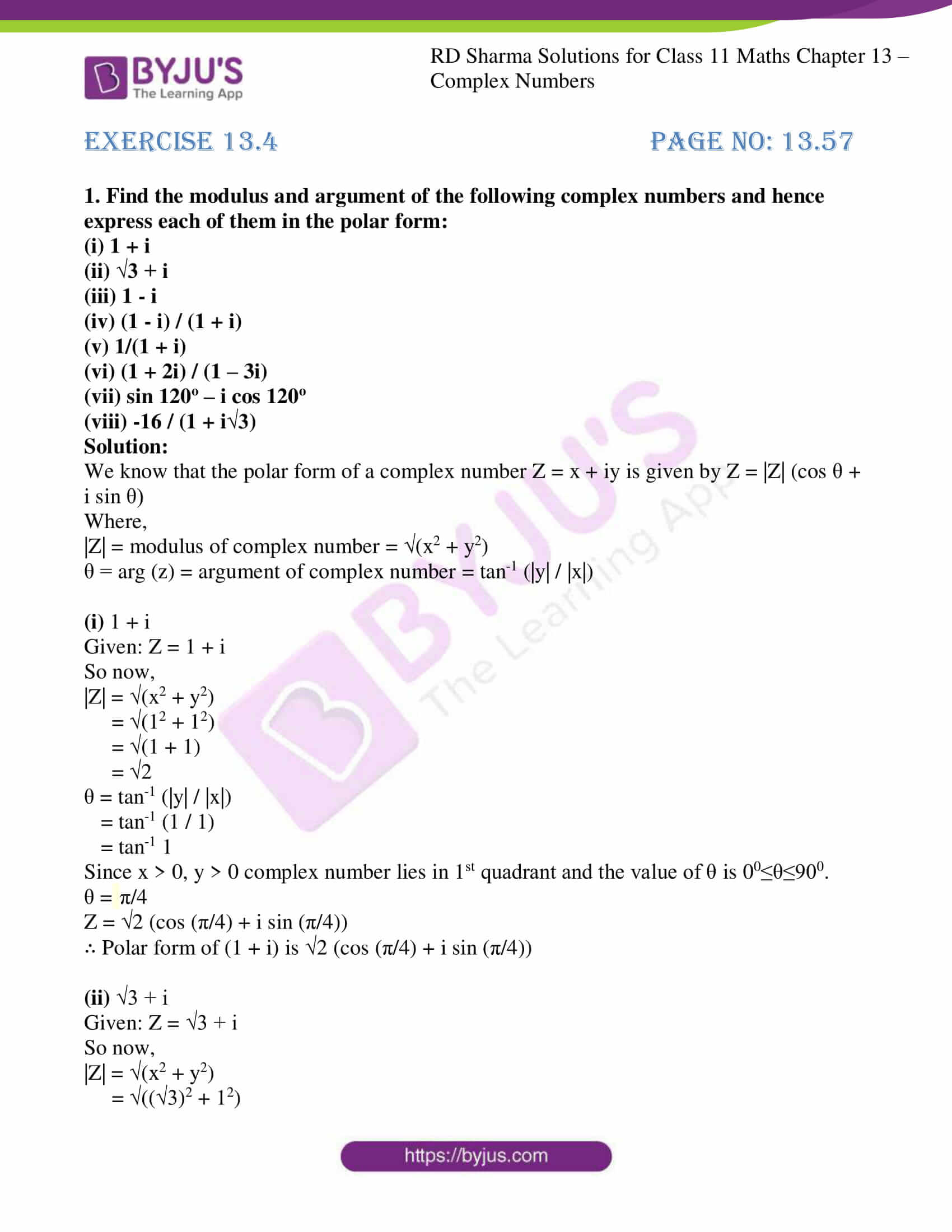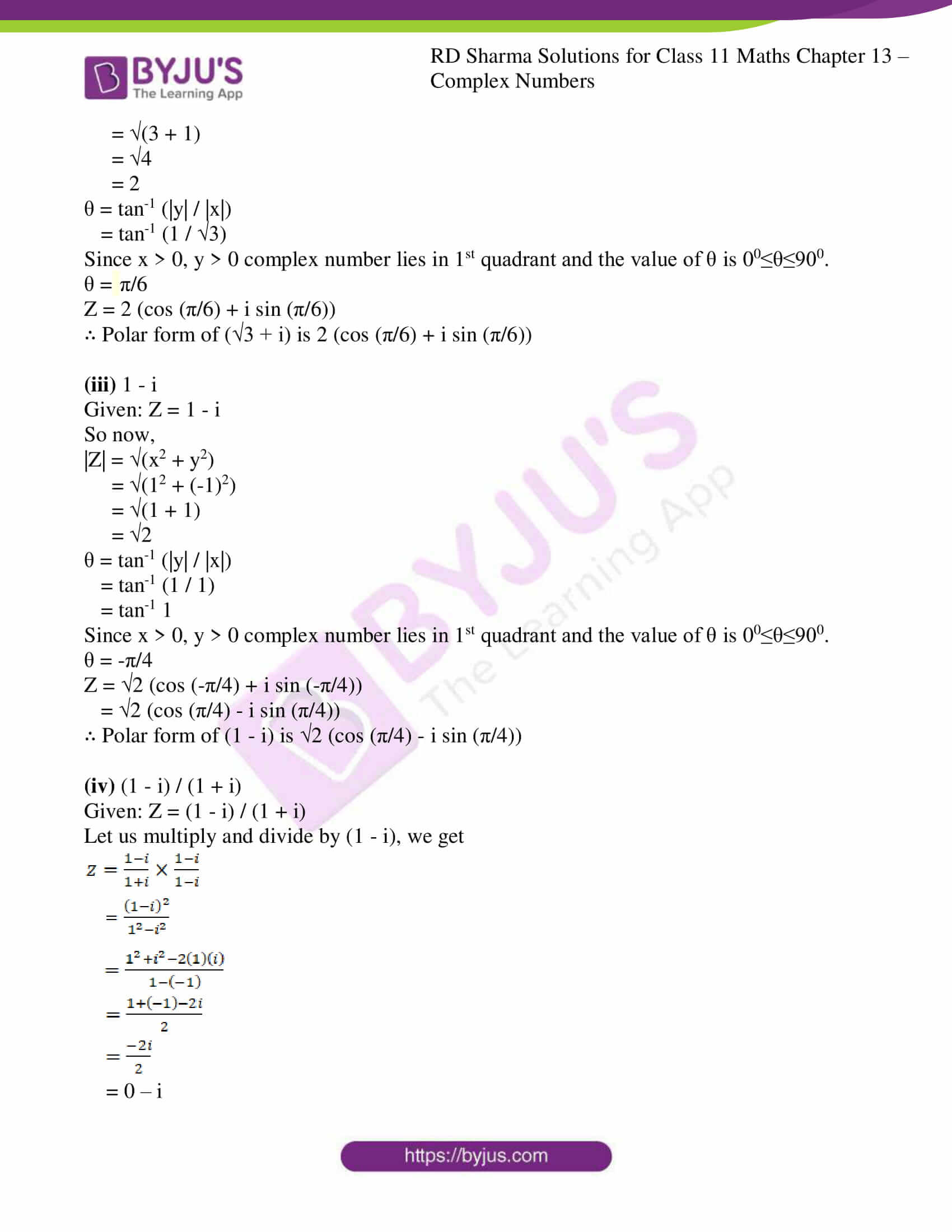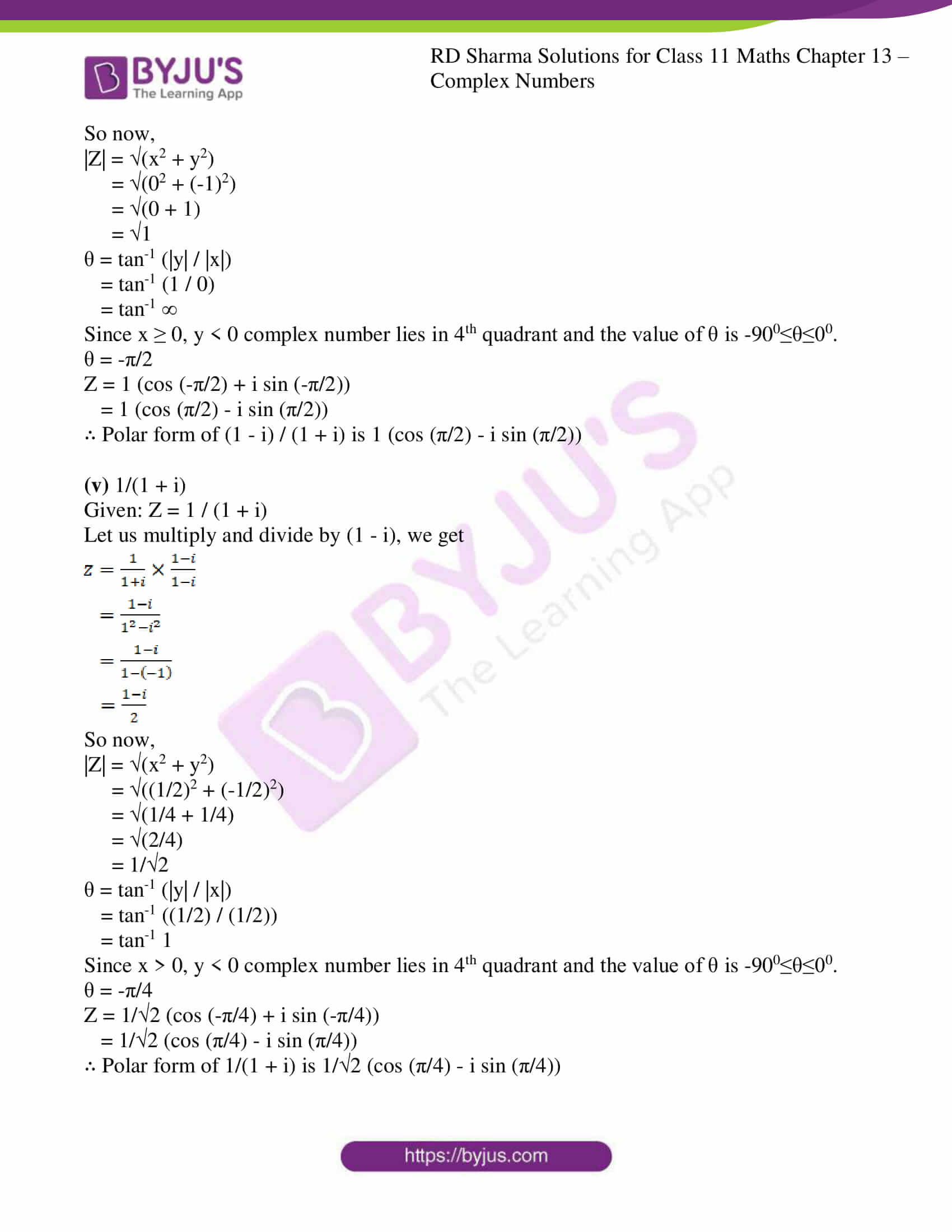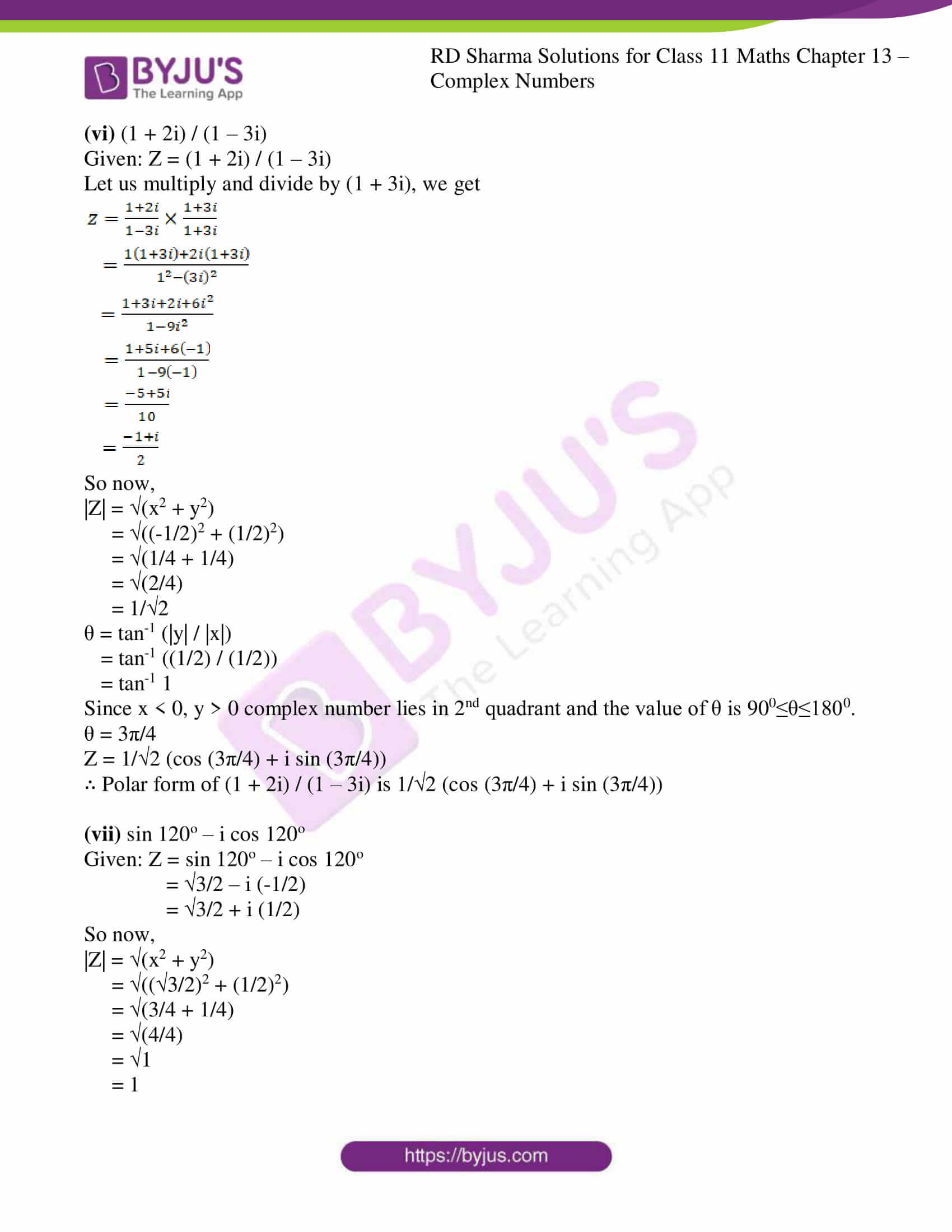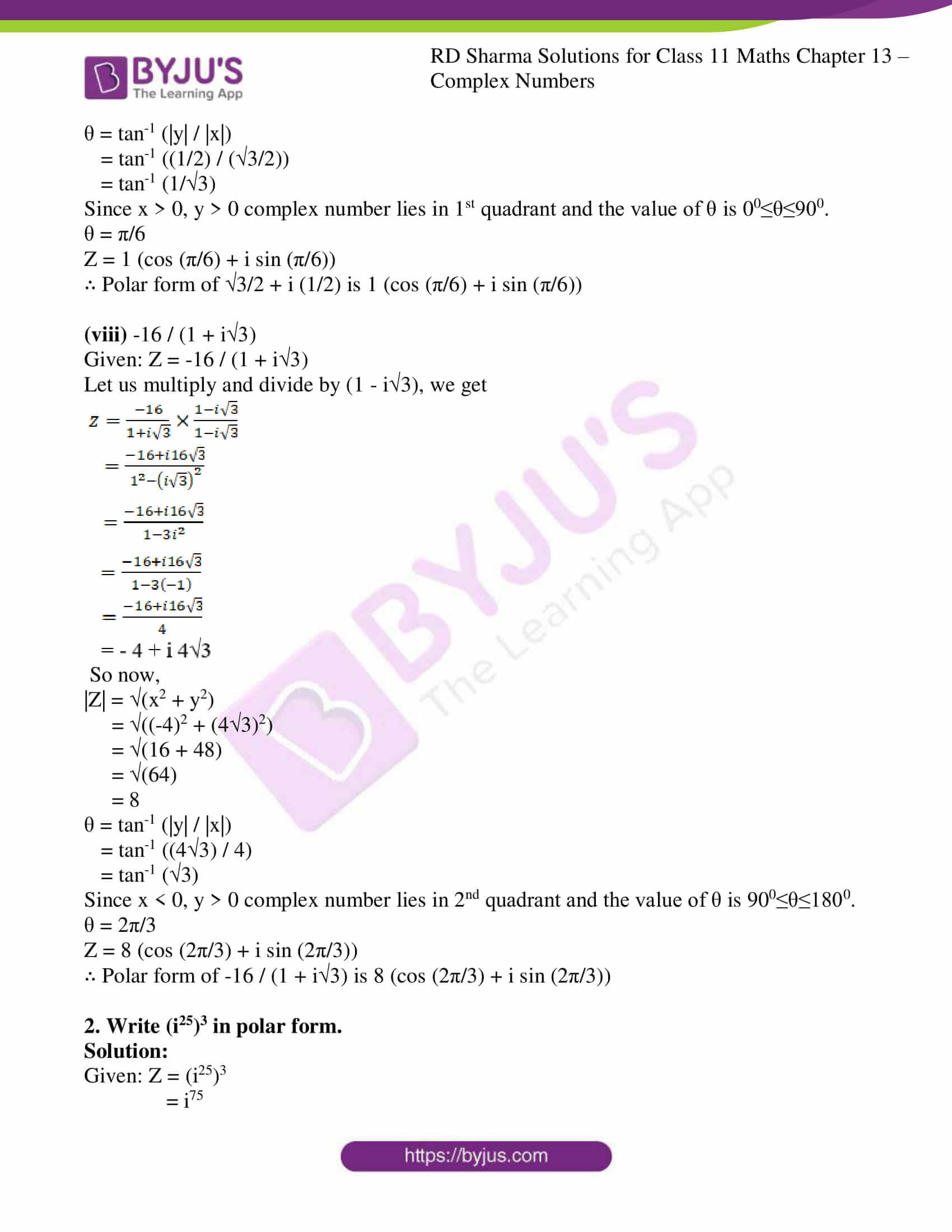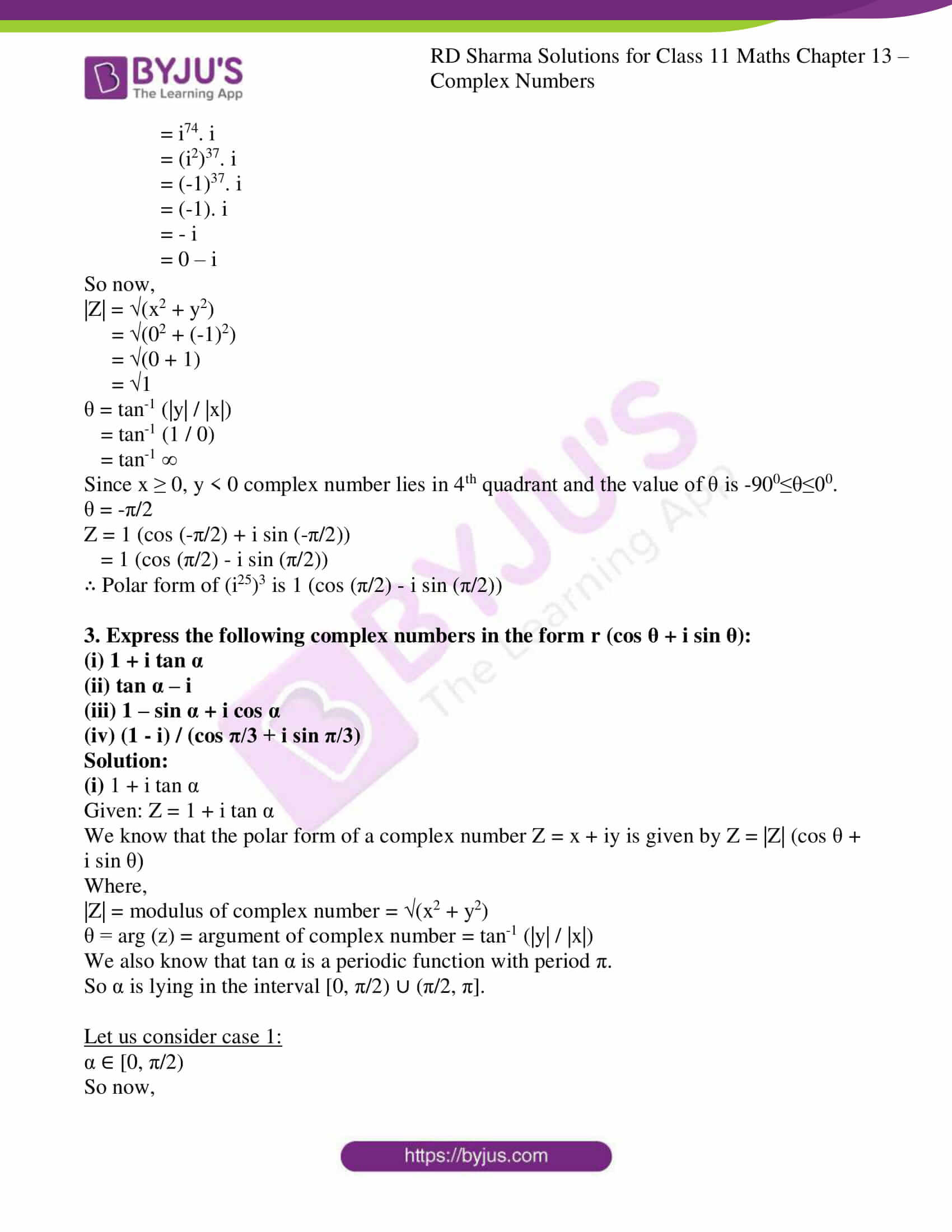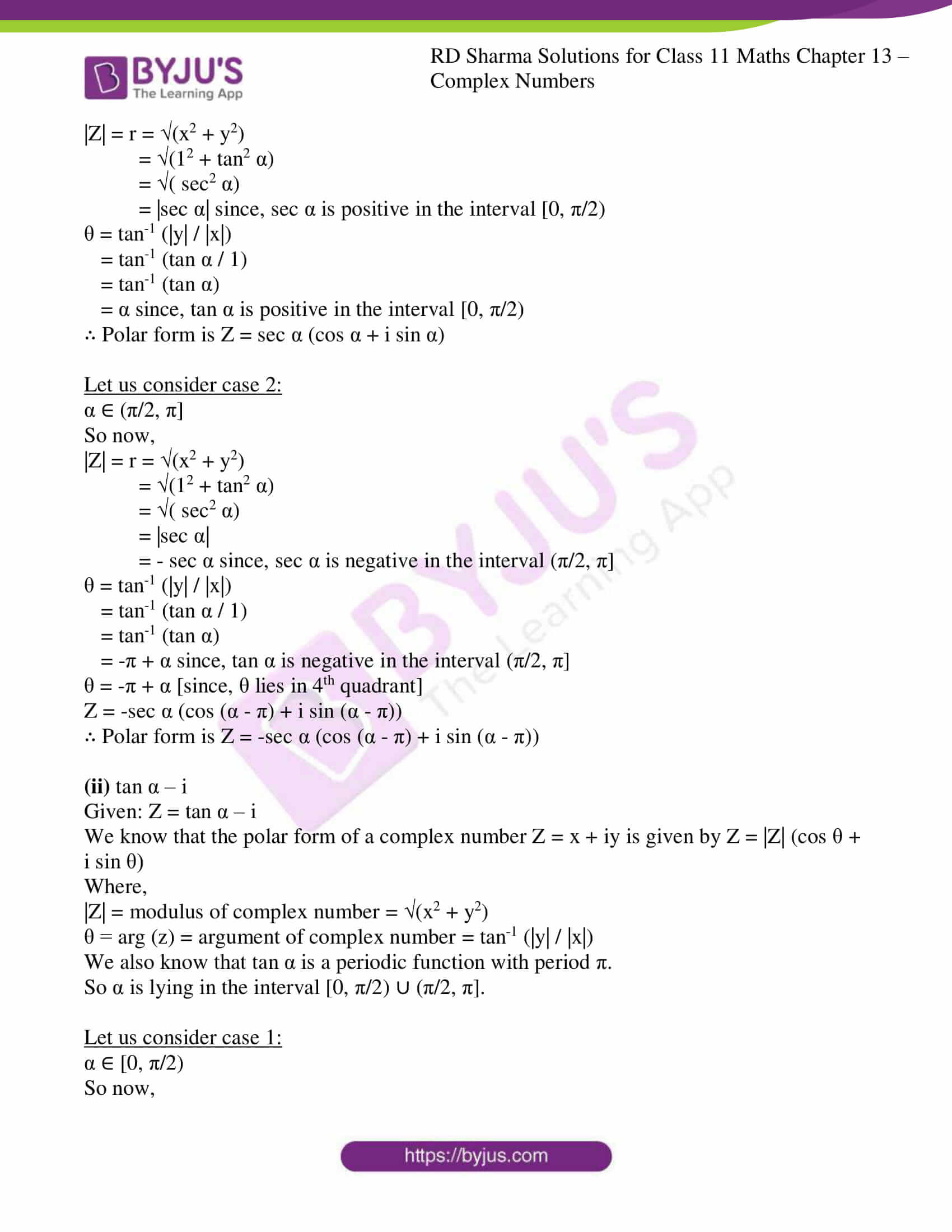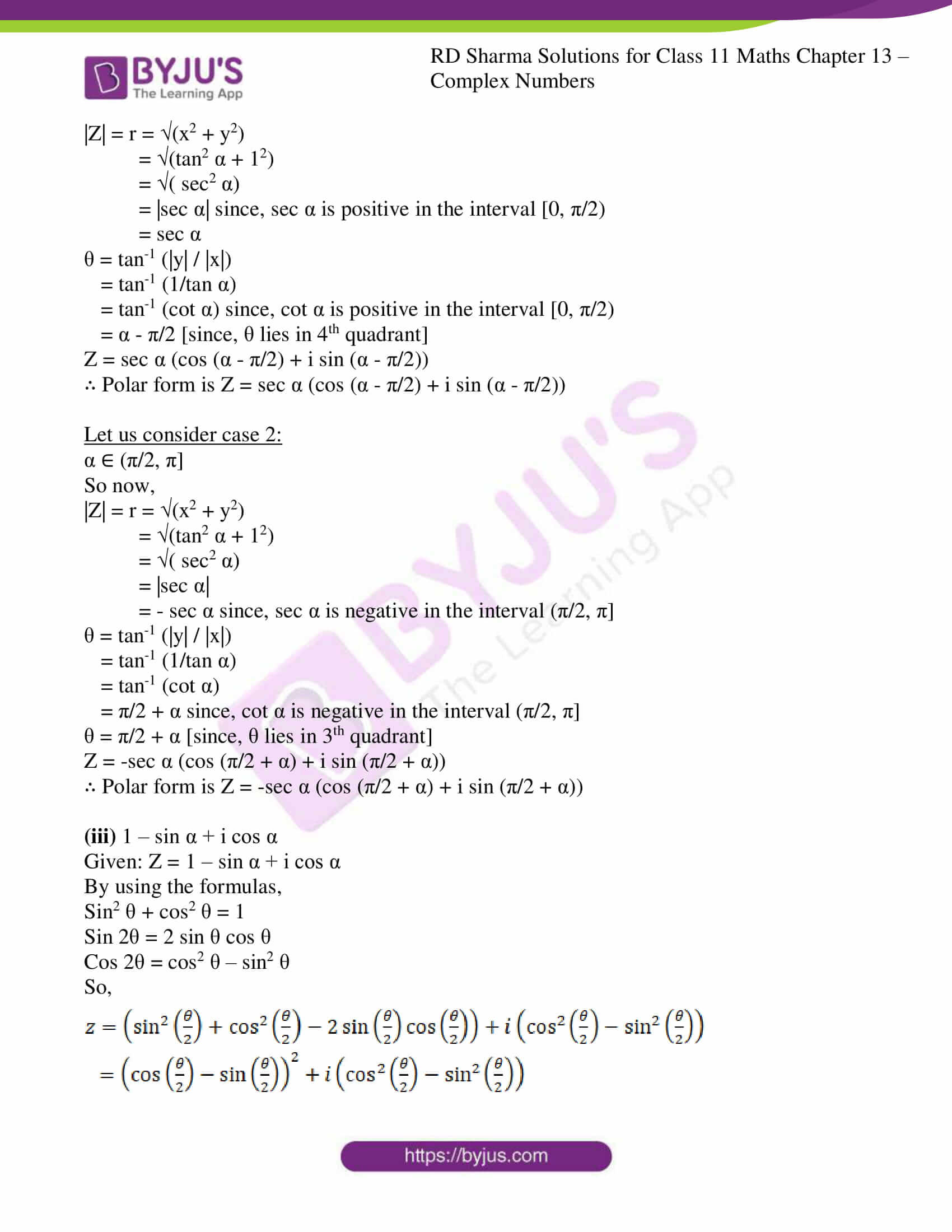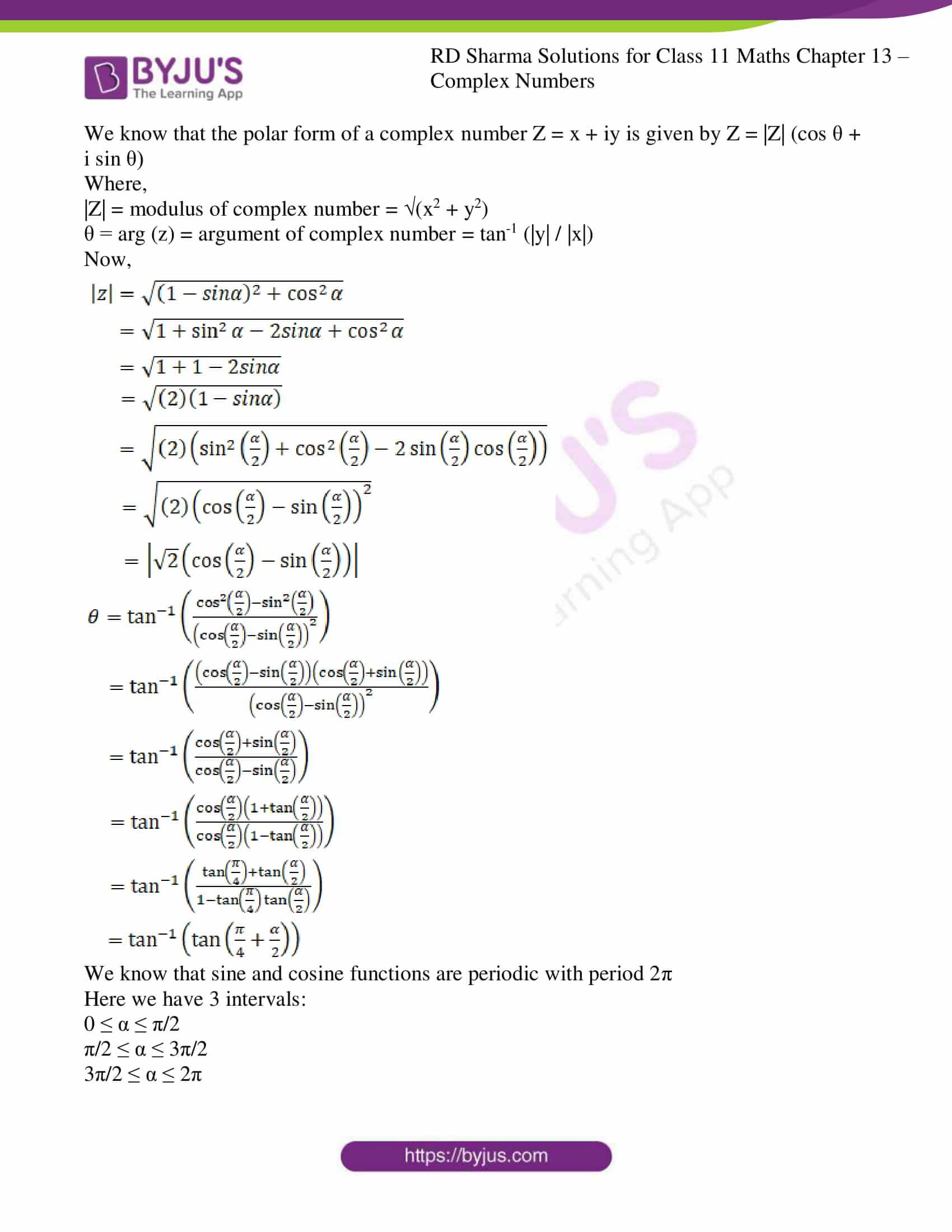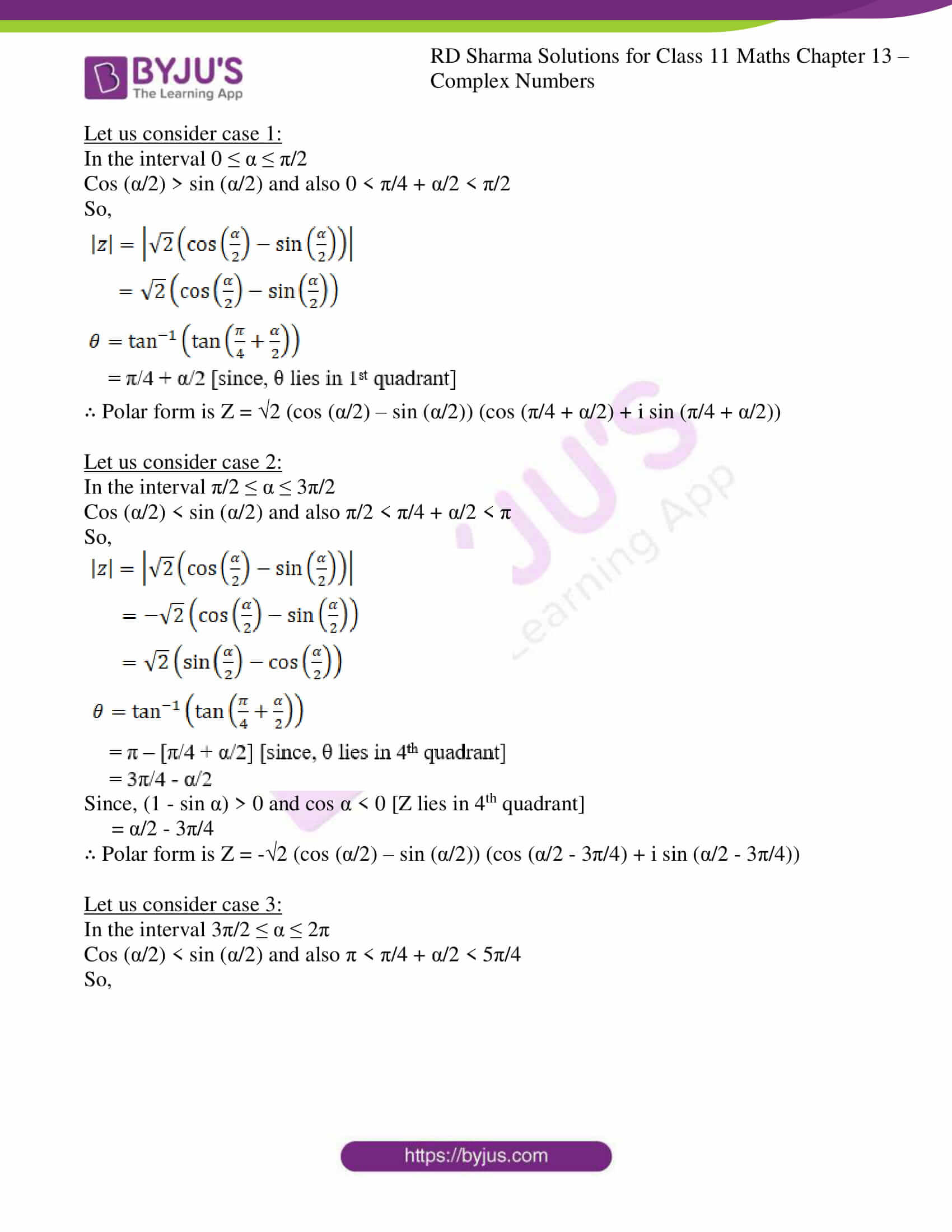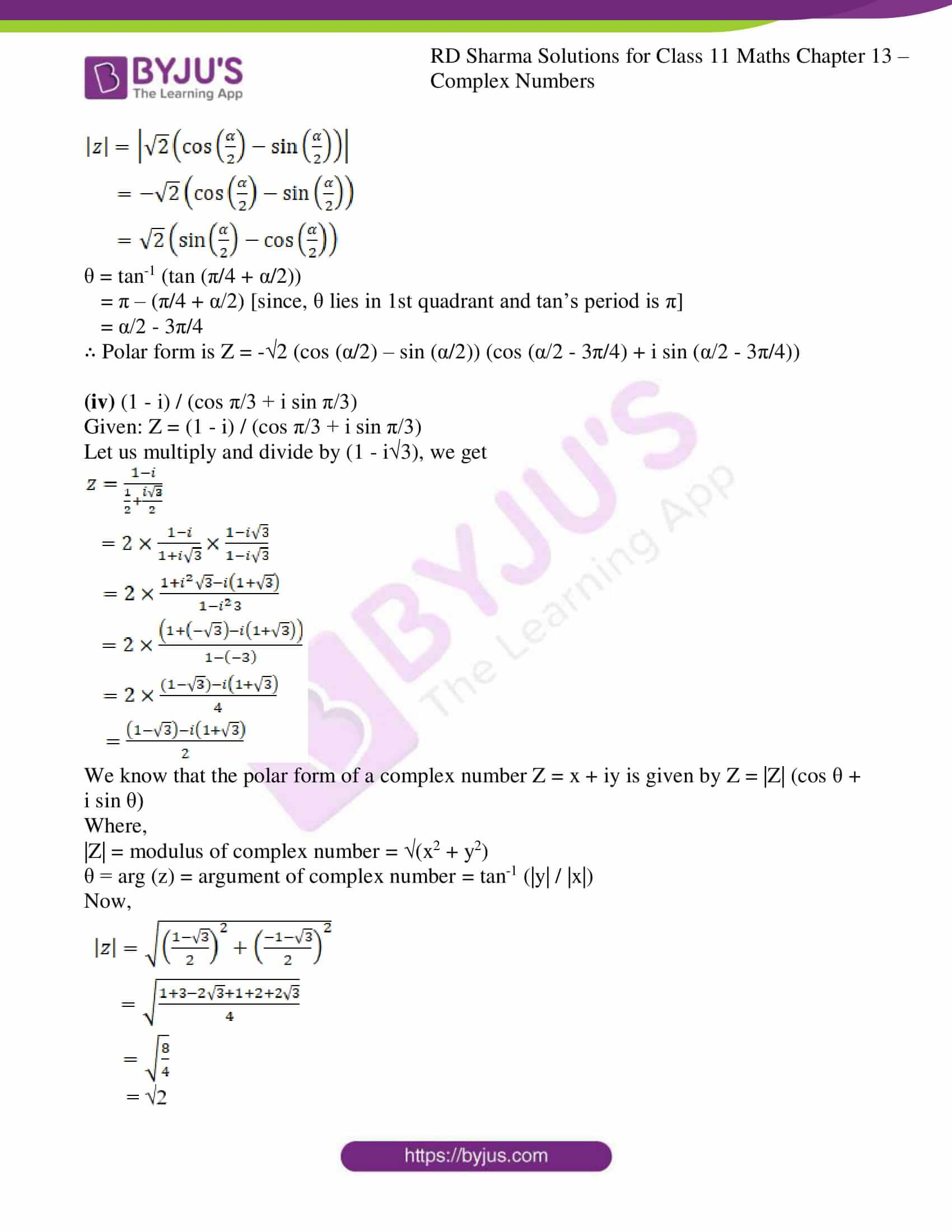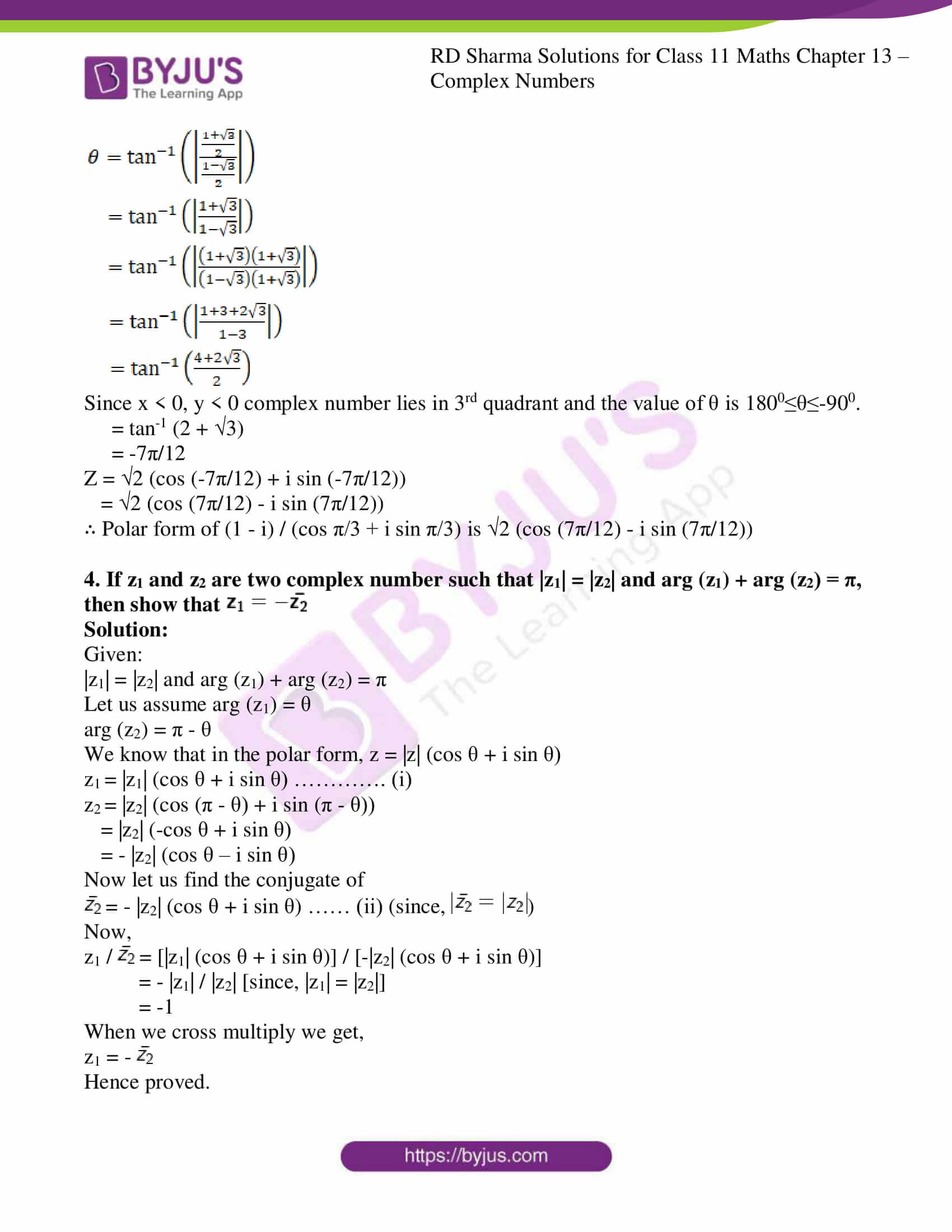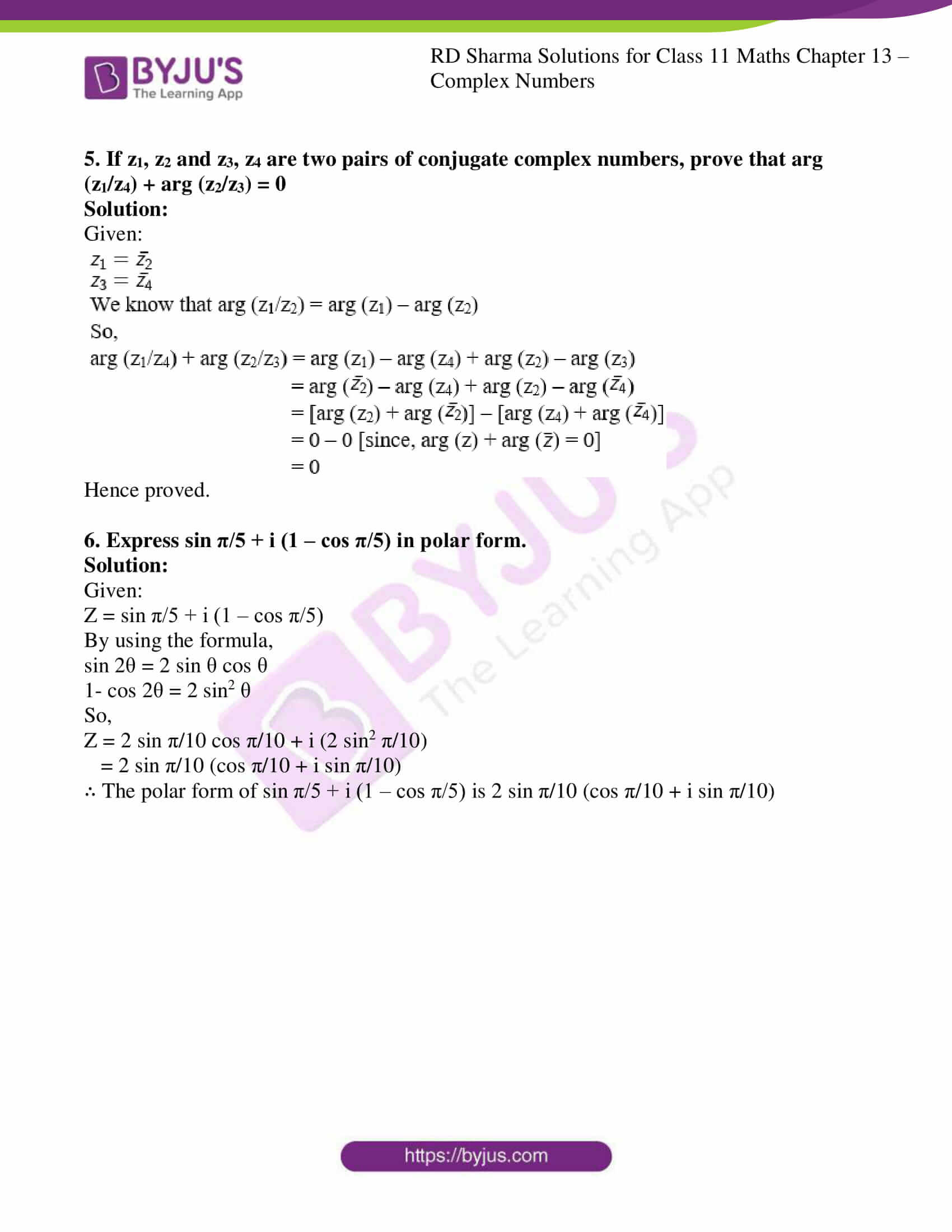### Access answers to RD Sharma Solutions for Class 11 Maths Exercise 13.4 Chapter 13 – Complex Numbers

1. Find the modulus and argument of the following complex numbers and hence express each of them in the polar form:

(i) 1 + i

(ii) √3 + i

(iii) 1 – i

(iv) (1 – i) / (1 + i)

(v) 1/(1 + i)

(vi) (1 + 2i) / (1 – 3i)

(vii) sin 120o – i cos 120o

(viii) -16 / (1 + i√3)

Solution:

We know that the polar form of a complex number Z = x + iy is given by Z = |Z| (cos θ + i sin θ)

Where,

|Z| = modulus of complex number = (x2 + y2)

θ = arg (z) = argument of complex number = tan-1 (|y| / |x|)

(i) 1 + i

Given: Z = 1 + i

So now,

|Z| = (x2 + y2)

= (12 + 12)

= (1 + 1)

= 2

θ = tan-1 (|y| / |x|)

= tan-1 (1 / 1)

= tan-1 1

Since x > 0, y > 0 complex number lies in 1st quadrant and the value of θ is 00≤θ≤900.

θ = π/4

Z = 2 (cos (π/4) + i sin (π/4))

∴ Polar form of (1 + i) is 2 (cos (π/4) + i sin (π/4))

(ii) √3 + i

Given: Z = √3 + i

So now,

|Z| = (x2 + y2)

= ((√3)2 + 12)

= (3 + 1)

= 4

= 2

θ = tan-1 (|y| / |x|)

= tan-1 (1 / √3)

Since x > 0, y > 0 complex number lies in 1st quadrant and the value of θ is 00≤θ≤900.

θ = π/6

Z = 2 (cos (π/6) + i sin (π/6))

∴ Polar form of (√3 + i) is 2 (cos (π/6) + i sin (π/6))

(iii) 1 – i

Given: Z = 1 – i

So now,

|Z| = (x2 + y2)

= (12 + (-1)2)

= (1 + 1)

= 2

θ = tan-1 (|y| / |x|)

= tan-1 (1 / 1)

= tan-1 1

Since x > 0, y > 0 complex number lies in 1st quadrant and the value of θ is 00≤θ≤900.

θ = -π/4

Z = 2 (cos (-π/4) + i sin (-π/4))

= 2 (cos (π/4) – i sin (π/4))

∴ Polar form of (1 – i) is 2 (cos (π/4) – i sin (π/4))

(iv) (1 – i) / (1 + i)

Given: Z = (1 – i) / (1 + i)

Let us multiply and divide by (1 – i), we get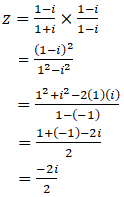= 0 – i

So now,

|Z| = (x2 + y2)

= (02 + (-1)2)

= (0 + 1)

= 1

θ = tan-1 (|y| / |x|)

= tan-1 (1 / 0)

= tan-1

Since x ≥ 0, y < 0 complex number lies in 4th quadrant and the value of θ is -900≤θ≤00.

θ = -π/2

Z = 1 (cos (-π/2) + i sin (-π/2))

= 1 (cos (π/2) – i sin (π/2))

∴ Polar form of (1 – i) / (1 + i) is 1 (cos (π/2) – i sin (π/2))

(v) 1/(1 + i)

Given: Z = 1 / (1 + i)

Let us multiply and divide by (1 – i), we get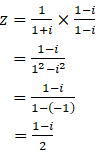So now,

|Z| = (x2 + y2)

= ((1/2)2 + (-1/2)2)

= (1/4 + 1/4)

= (2/4)

= 1/2

θ = tan-1 (|y| / |x|)

= tan-1 ((1/2) / (1/2))

= tan-1 1

Since x > 0, y < 0 complex number lies in 4th quadrant and the value of θ is -900≤θ≤00.

θ = -π/4

Z = 1/2 (cos (-π/4) + i sin (-π/4))

= 1/2 (cos (π/4) – i sin (π/4))

∴ Polar form of 1/(1 + i) is 1/2 (cos (π/4) – i sin (π/4))

(vi) (1 + 2i) / (1 – 3i)

Given: Z = (1 + 2i) / (1 – 3i)

Let us multiply and divide by (1 + 3i), we get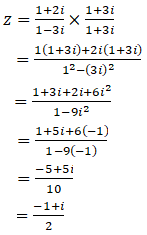So now,

|Z| = (x2 + y2)

= ((-1/2)2 + (1/2)2)

= (1/4 + 1/4)

= (2/4)

= 1/2

θ = tan-1 (|y| / |x|)

= tan-1 ((1/2) / (1/2))

= tan-1 1

Since x < 0, y > 0 complex number lies in 2nd quadrant and the value of θ is 900≤θ≤1800.

θ = 3π/4

Z = 1/2 (cos (3π/4) + i sin (3π/4))

∴ Polar form of (1 + 2i) / (1 – 3i) is 1/2 (cos (3π/4) + i sin (3π/4))

(vii) sin 120o – i cos 120o

Given: Z = sin 120o – i cos 120o

= 3/2 – i (-1/2)

= 3/2 + i (1/2)

So now,

|Z| = (x2 + y2)

= ((3/2)2 + (1/2)2)

= (3/4 + 1/4)

= (4/4)

= 1

= 1

θ = tan-1 (|y| / |x|)

= tan-1 ((1/2) / (3/2))

= tan-1 (1/3)

Since x > 0, y > 0 complex number lies in 1st quadrant and the value of θ is 00≤θ≤900.

θ = π/6

Z = 1 (cos (π/6) + i sin (π/6))

∴ Polar form of 3/2 + i (1/2) is 1 (cos (π/6) + i sin (π/6))

(viii) -16 / (1 + i√3)

Given: Z = -16 / (1 + i√3)

Let us multiply and divide by (1 – i3), we get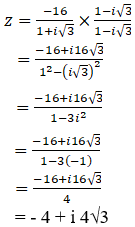So now,

|Z| = (x2 + y2)

= ((-4)2 + (43)2)

= (16 + 48)

= (64)

= 8

θ = tan-1 (|y| / |x|)

= tan-1 ((43) / 4)

= tan-1 (3)

Since x < 0, y > 0 complex number lies in 2nd quadrant and the value of θ is 900≤θ≤1800.

θ = 2π/3

Z = 8 (cos (2π/3) + i sin (2π/3))

∴ Polar form of -16 / (1 + i√3) is 8 (cos (2π/3) + i sin (2π/3))

2. Write (i25)3 in polar form.

Solution:

Given: Z = (i25)3

= i75

= i74. i

= (i2)37. i

= (-1)37. i

= (-1). i

= – i

= 0 – i

So now,

|Z| = (x2 + y2)

= (02 + (-1)2)

= (0 + 1)

= 1

θ = tan-1 (|y| / |x|)

= tan-1 (1 / 0)

= tan-1

Since x ≥ 0, y < 0 complex number lies in 4th quadrant and the value of θ is -900≤θ≤00.

θ = -π/2

Z = 1 (cos (-π/2) + i sin (-π/2))

= 1 (cos (π/2) – i sin (π/2))

∴ Polar form of (i25)3 is 1 (cos (π/2) – i sin (π/2))

3. Express the following complex numbers in the form r (cos θ + i sin θ):

(i) 1 + i tan α

(ii) tan α – i

(iii) 1 – sin α + i cos α

(iv) (1 – i) / (cos π/3 + i sin π/3)

Solution:

(i) 1 + i tan α

Given: Z = 1 + i tan α

We know that the polar form of a complex number Z = x + iy is given by Z = |Z| (cos θ + i sin θ)

Where,

|Z| = modulus of complex number = (x2 + y2)

θ = arg (z) = argument of complex number = tan-1 (|y| / |x|)

We also know that tan α is a periodic function with period π.

So α is lying in the interval [0, π/2) ∪ (π/2, π].

Let us consider case 1:

α ∈ [0, π/2)

So now,

|Z| = r = (x2 + y2)

= (12 + tan2 α)

= ( sec2 α)

= |sec α| since, sec α is positive in the interval [0, π/2)

θ = tan-1 (|y| / |x|)

= tan-1 (tan α / 1)

= tan-1 (tan α)

= α since, tan α is positive in the interval [0, π/2)

∴ Polar form is Z = sec α (cos α + i sin α)

Let us consider case 2:

α ∈ (π/2, π]

So now,

|Z| = r = (x2 + y2)

= (12 + tan2 α)

= ( sec2 α)

= |sec α|

= – sec α since, sec α is negative in the interval (π/2, π]

θ = tan-1 (|y| / |x|)

= tan-1 (tan α / 1)

= tan-1 (tan α)

= -π + α since, tan α is negative in the interval (π/2, π]

θ = -π + α [since, θ lies in 4th quadrant]

Z = -sec α (cos (α – π) + i sin (α – π))

∴ Polar form is Z = -sec α (cos (α – π) + i sin (α – π))

(ii) tan α – i

Given: Z = tan α – i

We know that the polar form of a complex number Z = x + iy is given by Z = |Z| (cos θ + i sin θ)

Where,

|Z| = modulus of complex number = (x2 + y2)

θ = arg (z) = argument of complex number = tan-1 (|y| / |x|)

We also know that tan α is a periodic function with period π.

So α is lying in the interval [0, π/2) ∪ (π/2, π].

Let us consider case 1:

α ∈ [0, π/2)

So now,

|Z| = r = (x2 + y2)

= (tan2 α + 12)

= ( sec2 α)

= |sec α| since, sec α is positive in the interval [0, π/2)

= sec α

θ = tan-1 (|y| / |x|)

= tan-1 (1/tan α)

= tan-1 (cot α) since, cot α is positive in the interval [0, π/2)

= α – π/2 [since, θ lies in 4th quadrant]

Z = sec α (cos (α – π/2) + i sin (α – π/2))

∴ Polar form is Z = sec α (cos (α – π/2) + i sin (α – π/2))

Let us consider case 2:

α ∈ (π/2, π]

So now,

|Z| = r = (x2 + y2)

= (tan2 α + 12)

= ( sec2 α)

= |sec α|

= – sec α since, sec α is negative in the interval (π/2, π]

θ = tan-1 (|y| / |x|)

= tan-1 (1/tan α)

= tan-1 (cot α)

= π/2 + α since, cot α is negative in the interval (π/2, π]

θ = π/2 + α [since, θ lies in 3th quadrant]

Z = -sec α (cos (π/2 + α) + i sin (π/2 + α))

∴ Polar form is Z = -sec α (cos (π/2 + α) + i sin (π/2 + α))

(iii) 1 – sin α + i cos α

Given: Z = 1 – sin α + i cos α

By using the formulas,

Sin2 θ + cos2 θ = 1

Sin 2θ = 2 sin θ cos θ

Cos 2θ = cos2 θ – sin2 θ

So,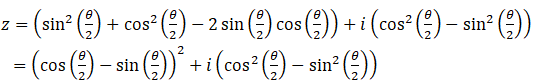We know that the polar form of a complex number Z = x + iy is given by Z = |Z| (cos θ + i sin θ)

Where,

|Z| = modulus of complex number = (x2 + y2)

θ = arg (z) = argument of complex number = tan-1 (|y| / |x|)

Now,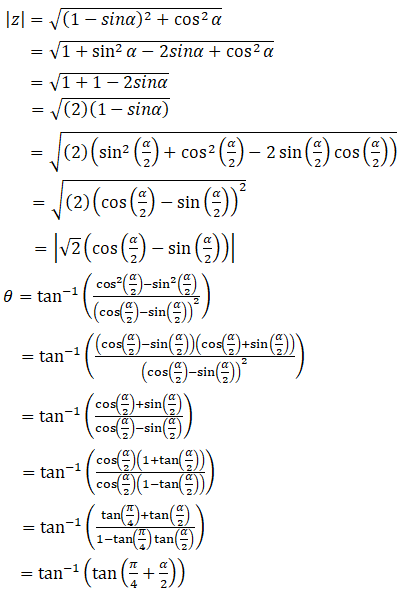We know that sine and cosine functions are periodic with period 2π

Here we have 3 intervals:

0 ≤ α ≤ π/2

π/2 ≤ α ≤ 3π/2

3π/2 ≤ α ≤ 2π

Let us consider case 1:

In the interval 0 ≤ α ≤ π/2

Cos (α/2) > sin (α/2) and also 0 < π/4 + α/2 < π/2

So,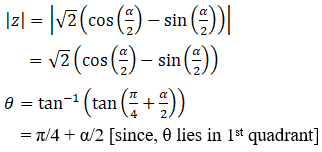∴ Polar form is Z = 2 (cos (α/2) – sin (α/2)) (cos (π/4 + α/2) + i sin (π/4 + α/2))

Let us consider case 2:

In the interval π/2 ≤ α ≤ 3π/2

Cos (α/2) < sin (α/2) and also π/2 < π/4 + α/2 < π

So,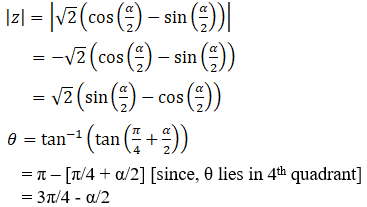Since, (1 – sin α) > 0 and cos α < 0 [Z lies in 4th quadrant]

= α/2 – 3π/4

∴ Polar form is Z = –2 (cos (α/2) – sin (α/2)) (cos (α/2 – 3π/4) + i sin (α/2 – 3π/4))

Let us consider case 3:

In the interval 3π/2 ≤ α ≤ 2π

Cos (α/2) < sin (α/2) and also π < π/4 + α/2 < 5π/4

So,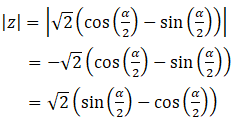θ = tan-1 (tan (π/4 + α/2))

= π – (π/4 + α/2) [since, θ lies in 1st quadrant and tan’s period is π]

= α/2 – 3π/4

∴ Polar form is Z = –2 (cos (α/2) – sin (α/2)) (cos (α/2 – 3π/4) + i sin (α/2 – 3π/4))

(iv) (1 – i) / (cos π/3 + i sin π/3)

Given: Z = (1 – i) / (cos π/3 + i sin π/3)

Let us multiply and divide by (1 – i3), we get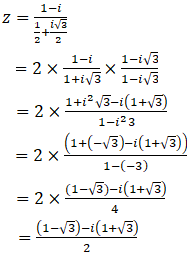We know that the polar form of a complex number Z = x + iy is given by Z = |Z| (cos θ + i sin θ)

Where,

|Z| = modulus of complex number = (x2 + y2)

θ = arg (z) = argument of complex number = tan-1 (|y| / |x|)

Now,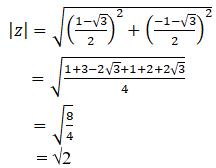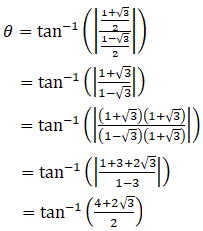Since x < 0, y < 0 complex number lies in 3rd quadrant and the value of θ is 1800≤θ≤-900.

= tan-1 (2 + 3)

= -7π/12

Z = 2 (cos (-7π/12) + i sin (-7π/12))

= 2 (cos (7π/12) – i sin (7π/12))

∴ Polar form of (1 – i) / (cos π/3 + i sin π/3) is 2 (cos (7π/12) – i sin (7π/12))

4. If z1 and z2 are two complex number such that |z1| = |z2| and arg (z1) + arg (z2) = π, then show that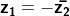Solution:

Given:

|z1| = |z2| and arg (z1) + arg (z2) = π

Let us assume arg (z1) = θ

arg (z2) = π – θ

We know that in the polar form, z = |z| (cos θ + i sin θ)

z1 = |z1| (cos θ + i sin θ) …………. (i)

z2 = |z2| (cos (π – θ) + i sin (π – θ))

= |z2| (-cos θ + i sin θ)

= – |z2| (cos θ – i sin θ)

Now let us find the conjugate of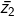= – |z2| (cos θ + i sin θ) …… (ii) (since,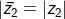)

Now,

z1 /= [|z1| (cos θ + i sin θ)] / [-|z2| (cos θ + i sin θ)]

= – |z1| / |z2| [since, |z1| = |z2|]

= -1

When we cross multiply we get,

z1 = –Hence proved.

5. If z1, z2 and z3, z4 are two pairs of conjugate complex numbers, prove that arg (z1/z4) + arg (z2/z3) = 0

Solution:

Given: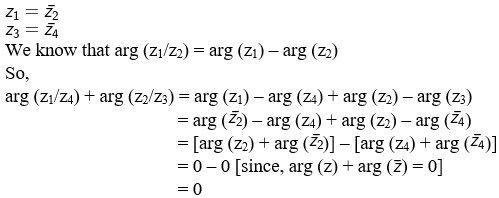Hence proved.

6. Express sin π/5 + i (1 – cos π/5) in polar form.

Solution:

Given:

Z = sin π/5 + i (1 – cos π/5)

By using the formula,

sin 2θ = 2 sin θ cos θ

1- cos 2θ = 2 sin2 θ

So,

Z = 2 sin π/10 cos π/10 + i (2 sin2 π/10)

= 2 sin π/10 (cos π/10 + i sin π/10)

∴ The polar form of sin π/5 + i (1 – cos π/5) is 2 sin π/10 (cos π/10 + i sin π/10)